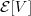9.7 COMPLETE STATISTIC

Although T = [X(1), X(N)]T is minimal sufficient for θ of the uniform distribution in Example 9.12, and together they contain as much information about θ as the original samples, it turns out they also contain information that is not relevant for estimating θ. This irrelevant information is called ancillary, and any component of a minimal sufficient statistic that is ancillary is called an ancillary statistic. A minimal sufficient statistic that has no ancillary component is called a complete minimal sufficient statistic. We provide two definitions of an ancillary statistic.

Definition: Ancillary Statistic Statistic V is ancillary if its distribution is independent of θ.

An ancillary statistic does not contain any information about parameter θ. This is essentially the opposite property of a sufficient statistic which contains the same information about θ as the original samples.

Definition: First-Order Ancillary Statistic Statistic V is first-order ancillary ifis independent of θ.

An ancillary statistic is also first-order ancillary: if its distribution is independent of θ, then its expectation does not depend on θ.

Example 9.16. For the uniform pdf, observe that the statistic V = X(N)X(1) is first-order ancillary because its mean does not depend on θ:

Get Probability, Random Variables, and Random Processes: Theory and Signal Processing Applications now with the O’Reilly learning platform.

O’Reilly members experience books, live events, courses curated by job role, and more from O’Reilly and nearly 200 top publishers.